### Model Fitting Statistics

Suppose the model containsexplanatory effects. For theth observation, let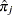be the estimated probability of the observed response. The three criteria displayed by the SURVEYLOGISTIC  procedure are calculated as follows:

•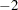log likelihood: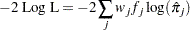where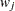and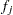are the weight and frequency values, respectively, of theth observation. For binary response models that use the events/trials syntax, this is equivalent to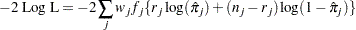where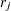is the number of events,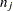is the number of trials, andis the estimated event probability.

• Akaike information criterion: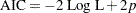whereis the number of parameters in the model. For cumulative response models,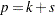, whereis the total number of response levels minus one, andis the number of explanatory effects. For the generalized logit model,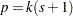.

• Schwarz criterion: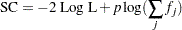whereis the number of parameters in the model. For cumulative response models,, whereis the total number of response levels minus one, andis the number of explanatory effects. For the generalized logit model,.

The2 log likelihood statistic has a chi-square distribution under the null hypothesis (that all the explanatory effects in the model are zero), and the procedure produces a-value for this statistic. The AIC and SC statistics give two different ways of adjusting thelog likelihood statistic for the number of terms in the model and the number of observations used.